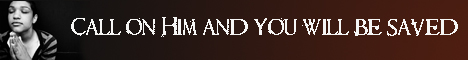Note:  Do not rely on this information. It is very old.

# Hexagon

Hexagon, in geometry, is a six-sided figure.

A regular hexagon has six equal sides, consecutive pairs of which contain six equal angles. The opposite sides of a regular hexagon are parallel, and can only be regarded as meeting at an infinite distance. The three pairs of opposite sides of any hexagon inscribed in any conic section intersect in three points which will always lie in a line. This is Pascal's theorem. Further, the three diagonals of a regular hexagon - i.e. the lines joining opposite angular points - pass through one point. If any hexagon be circumscribed about any conic the three diagonals will also be found to intersect at a point. This is Brianchon's theorem. [Duality, Principle of.]